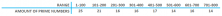Smartick is an advanced online program that teaches kids math and coding in only 15 min. a day

Dec24

# Some Prime Number Curiosities

For this entry, we are going to learn some surprising facts about prime numbers. We would recommend reading our post about prime numbers and composite numbers first, to refresh your knowledge about these numbers.

Are you ready to move on? Perfect!

I’m sure that you know it is possible to write some numbers as products of others, in other words, they can be broken down:For example, we can write: 18 = 2 x 3 x 3

However, this does not happen with all numbers. There are some numbers that exist which are impossible to break down.

These numbers are 23, 5, 7, 11…in other words, the prime numbers!

## The equivalent definition of prime number

As you know, when a number is only divisible by itself and the number one it is considered to be a prime number. However, this definition is hiding a secret which is… what about number 1? Is it prime? The answer is no. The key here is that in order for a number to be prime it has to be divisible by itself and one. Both aspects. To avoid this complication, mathematicians use the definition of equivalent primary numbers.

We say a number is a prime number if it has exactly two divisors.

## The fundamental theorem of arithmetic

Now that we clearly understand what a prime number is, we are going to look at some important mathematical findings. Earlier, we wrote that 18 is a product of the prime numbers 2 and 3. Could we solve this differently? The answer is no. This is not only true for the number 18, but for any number that you can think of. This is known as the Fundamental Theorem of Arithmetic and it is possibly one of the most important findings in all of mathematics.

Any integer greater than 1 is uniquely expressed as a product of prime numbers.

## How many prime numbers exist?

Unfortunately, there is not a formula that allows us to easily identify all the prime numbers. As a result of this, throughout history, mathematicians have had to develop their own methods to find them. Another challenge that mathematicians face is how to distribute prime numbers. In fact, the amount of prime numbers in a particular range varies. Take a look at the following table.Infinite prime numbers exist.

The reason for demonstrating this is very simple. Suppose the amount of prime numbers wasn’t infinite, then one would be greater than all of them. Let’s call that prime number P. Then, we create another number, we’ll call it Q, in the following way:

Q = (2 x 3 x 5 x 7 x 11 x … x P) + 1

This new number is the result of multiplying all the prime numbers until the last one and then adding 1. As it turns out, Q is not divisible by any prime number because the remainder of dividing it by any of them is 1. Try dividing 31 by 2, 3, and 5 and see for yourself! Therefore, Q is only divisible by itself and one, in other words, it’s prime! But clearly, Q is greater than P.

Therefore, we have found a prime number that is greater than all prime numbers. Since this is contradictory, the conclusion is that a prime number greater than all of them cannot exist because an infinite amount of prime numbers exists.

I hope that this post has helped you learn more about prime numbers. If you want to keep learning, join the Smartick community and try it for free.